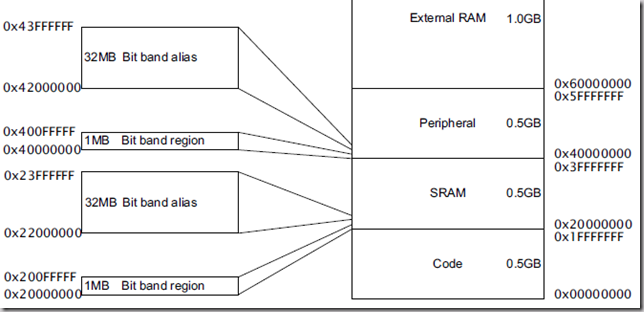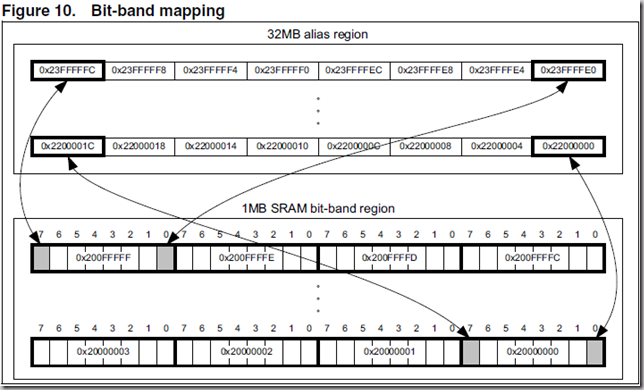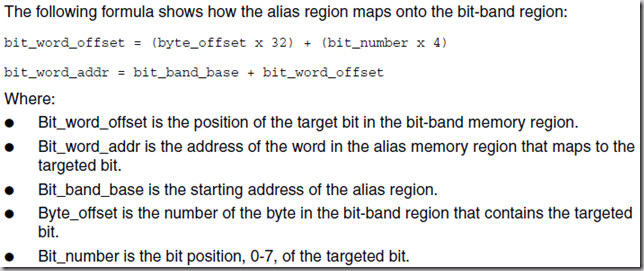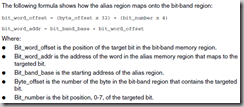## 从STM32的位带操作重谈嵌入式中寻址与对齐的理解

【@.1 从位带操作开始】

@-> PIN0 |= (1<<3);

@-> PIN0 &= ~(1<<5);

@-> PIN0BIT3 = 1;   //等同于PIN0 |= （1<<3)， 这是由地址重映射保证的。【@.2 字，字节，半字与寻址方式】

@-> 2^10=1024=1K

@-> 2^20=1024*1024=1M

@-> 2^30=1024*1024*1024=1G

@-> 2^32= 4 * 2^30=4*1G=4G

@-> 2^64=2^34 * 2^30=16G*G

/* General Purpose Input/Output (GPIO) */
#define IOPIN0         (*((volatile unsigned long *) 0xE0028000))
#define IOSET0         (*((volatile unsigned long *) 0xE0028004))
#define IODIR0         (*((volatile unsigned long *) 0xE0028008))
#define IOCLR0         (*((volatile unsigned long *) 0xE002800C))
#define IOPIN1         (*((volatile unsigned long *) 0xE0028010))
#define IOSET1         (*((volatile unsigned long *) 0xE0028014))
#define IODIR1         (*((volatile unsigned long *) 0xE0028018))
#define IOCLR1         (*((volatile unsigned long *) 0xE002801C))

【@.3 字节对齐，字节序 Endianness】

@-> #define IOPIN0 (*((volatile unsigned long *) 0xE0028000))

@-> (uint32*)0xE0028000  //当然也可以是unsigned int来代替uint32，都可以。

@-> (   *(  (uint32*)0xE0028000 )   )  //我故意多留了空格，目的是为了看得清楚。

@->   0xE0028000 ：0xDD

@->   0xE0028001 ：0xCC

@->   0xE0028002 ：0xBB

@->   0xE0028003 ：0xAA

【@.4 再看位带操作】

IN A NUTSHELL:

@-> 位带区（Bit band region）中的每一个bit均扩充到别名区（Bit band alias）上的一个字（Word），即4个字节（Byte），32个bit，因此总共1MB的位带区被扩充为32MB的别名区。

@-> 为什么每一个bit位要扩充为一个字（Word）而不是字节（Byte）？因为CPU进行常规操作都是以字（Word）为单位访问地址的。所以位带区的相邻一bit映射到别名区的地址增量是4，正好是4个字节（Byte），一个字（Word）。#define BITBAND(addr, bitnum) ((addr & 0xF0000000)+0x2000000+((addr &0xFFFFF)<<5)+(bitnum<<2))

#define PAout(n)   BIT_ADDR(GPIOA_ODR_Addr,n)  //OutPut

@-> PAout(4)=1

@.[FIN]      @.date->Dec 2, 2012      @.author->apollius

posted on 2012-12-02 20:15  apollius  阅读(2496)  评论(1编辑  收藏  举报